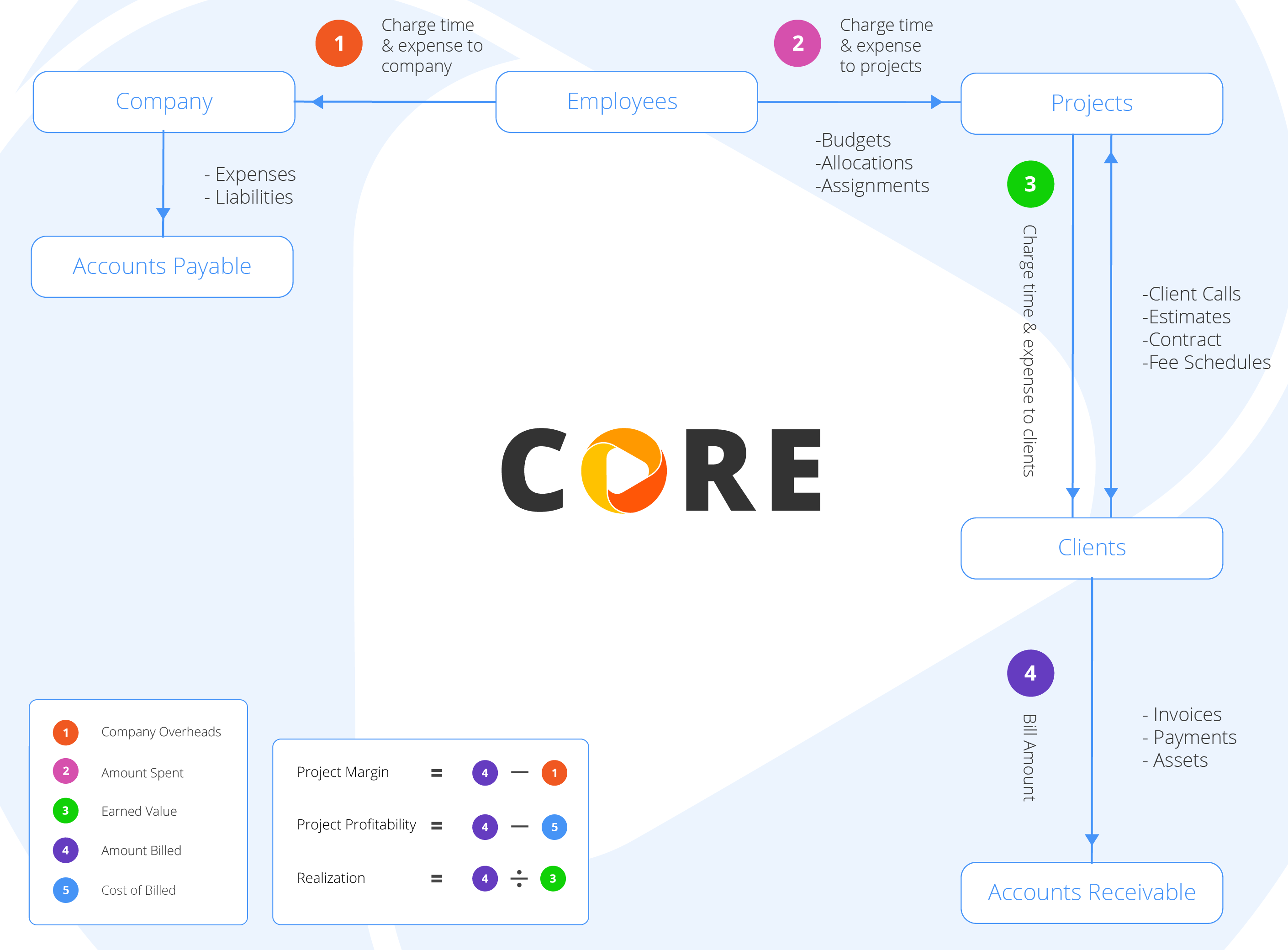## Who Charges Whom?

In order to understand the real profitability and cost of your projects, you need to understand who charges whom in CORE and how the calculations work. You can check out the flow diagram along with the example provided below:Cost Rate of an employee = \$50/hour

Bill Rate of the employee = \$100/hour

Actual Hours worked by the employee = 2 hours

Client Hours billed = 2 hours

Write-Up/Down = WUD = \$25

1. Company Overheads: Employees work on projects and in that process, they charge cost rates to the company on each project. These costs to the company are referred to as company overheads and are usually monitored by the company's owner or principal/partner. They are calculated as:

Company Overheads = Actual Hours x Cost Rate = 2 x 50 = \$100

2. Amount Spent: As employees work on projects, they charge each project at the default bill rates. These project costs are referred to as total amount spent and are usually monitored by the project manager (job costing). They include markups and taxes, if any, and are calculated as:

Amount Spent = Client Hours x Bill Rate = 2 x 100 = \$200

3. Earned Value: When you are billing the projects, the cumulative value of billable work done on them represents their earned value. These earnings are referred to as total invoice amount and exclude any adjustments such as taxes, retainers or discounts. It is calculated as:

Earned Value = Client Hours x Bill Rate = 2 x 100 = \$200

4. Amount Billed: Now the clients are charged for the work on the projects at the agreed upon or fee schedule rates according to the contract. These billings to the clients are referred to as total amount billed and are usually monitored by the company's owner or principal/partner. They are calculated as:

Amount Billed = (Client Hours x Bill Rate) + WUD = (2 x 100) + 25 = \$225

Based on the above costs and billings, you can calculate important metrics for the company as well as the projects.

Project Margin = Amount Billed - Company Overheads = 225 - 100 = \$125

Project Profitability = Amount Billed - Cost of Billed = 225 - 100* = \$125

Realization Rate = Amount Billed / Earned Value = 225 / 200 = 1.125

Realization % = Amount Billed / Earned Value x 100 = 225 / 200 x 100 = 112.5%

*where Cost of Billed = (Client Hours x Cost Rate) = (2 x \$50) = \$100